Next:8.4 Traveling Salesman ProblemUp:8.3 Minimum-Cost Spanning TreesPrevious:8.3.2 Prim's Algorithm

## 8.3.3 Kruskal's Algorithm

REF.
J.B. Kruskal. On the shortest spanning subtree of a graph and the traveling salesman problem. Proceedings of the American Mathematical Society, Volume 7, pp. 48-50, 1956.
• Complexity is O(elog e) where e is the number of edges. Can be made even more efficient by a proper choice of data structures.
• Greedy algorithm
• Algorithm:

•

Let G = (V, E) be the given graph, with | V| = n

{

Start with a graph T = (V,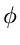) consisting of only the

vertices of G and no edges; /* This can be viewed as n

connected components, each vertex being one connected component */

Arrange E in the order of increasing costs;

for (i = 1, i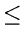n - 1, i + +)

{ Select the next smallest cost edge;

if (the edge connects two different connected components)

}

}

• At the end of the algorithm, we will be left with a single component that comprises all the vertices and this component will be an MST for G. Proof of Correctness of Kruskal's Algorithm

•

Theorem: Kruskal's algorithm finds a minimum spanning tree.

Proof: Let G = (V, E) be a weighted, connected graph. Let T be the edge set that is grown in Kruskal's algorithm. The proof is by mathematical induction on the number of edges in T.

• We show that if T is promising at any stage of the algorithm, then it is still promising when a new edge is added to it in Kruskal's algorithm
• When the algorithm terminates, it will happen that T gives a solution to the problem and hence an MST.
Basis: Tis promising since a weighted connected graph always has at least one MST.

Induction Step: Let T be promising just before adding a new edge e = (u, v). The edges T divide the nodes of G into one or more connected components. u and v will be in two different components. Let U be the set of nodes in the component that includes u. Note that

• U is a strict subset of V
• T is a promising set of edges such that no edge in T leaves U (since an edge T either has both ends in U or has neither end in U)
• e is a least cost edge that leaves U (since Kruskal's algorithm, being greedy, would have chosen e only after examining edges shorter than e)
The above three conditions are precisely like in the MST Lemma and hence we can conclude that the T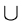{e} is also promising. When the algorithm stops, T gives not merely a spanning tree but a minimal spanning tree since it is promising.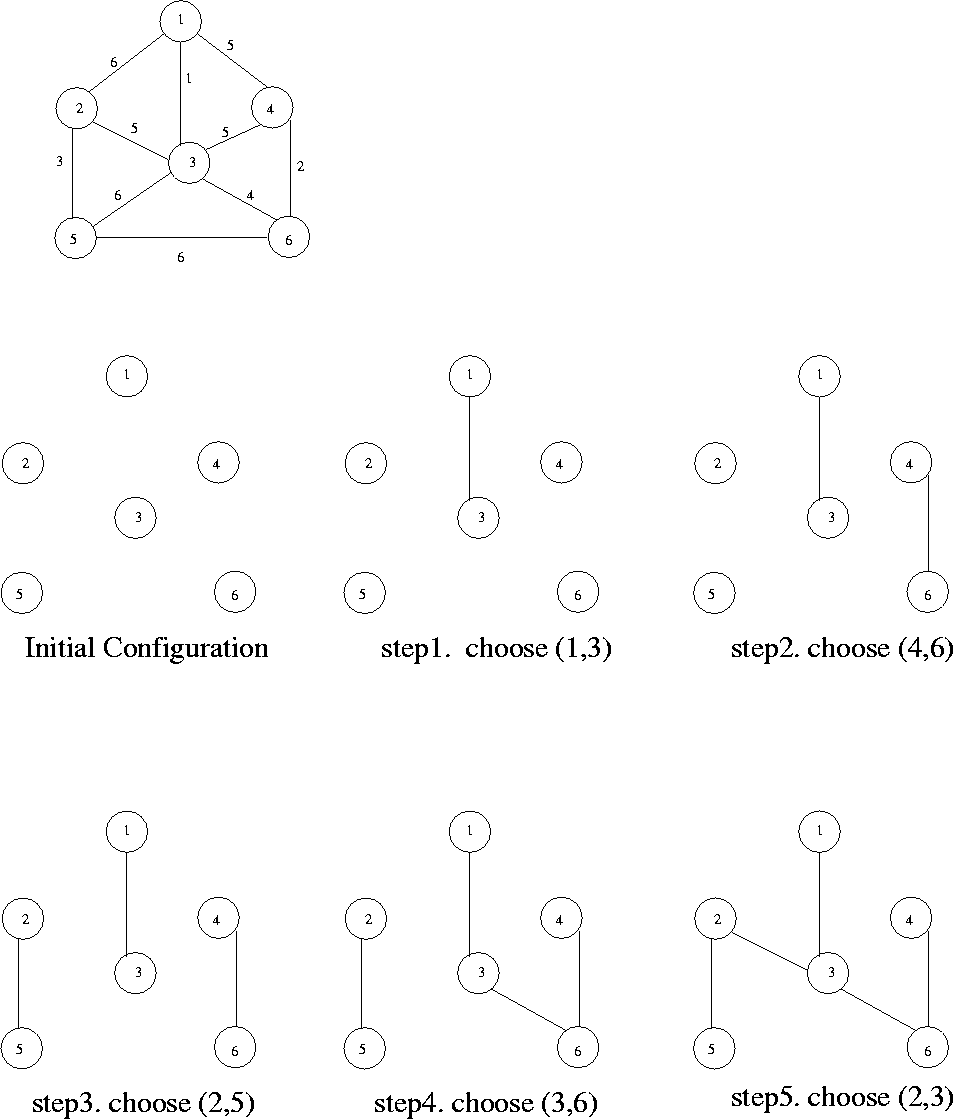• Program

void kruskal (vertex-set V; edge-set E; edge-set T)

int ncomp; /* current number of components */

priority-queue edges /* partially ordered tree */

mfset components; /* merge-find set data structure */

vertex u, v; edge e;

int nextcomp; /* name for new component */

int ucomp, vcomp; /* component names */

{

makenull (T); makenull (edges);

nextcomp = 0; ncomp = n;

for (v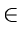V) /* initialize a component to have one vertex of V*/

{ nextcomp++ ;

initial (nextcomp, v, components);

}

for (eE)

insert (e, edges); /* initialize priority queue of edges */

while (ncomp > 1)

{

e = deletemin (edges);

let e = (u, v);

ucomp = find(u, components);

vcomp = find(v, components);

if (ucomp! = vcomp)

{

merge (ucomp, vcomp, components);

ncomp = ncomp - 1;

}

}

}

Implementation

• Choose a partially ordered tree for representing the sorted set of edges
• To represent connected components and interconnecting them, we need to implement:
• 1.
MERGE (A, B, C) . . . merge components A and B in C and call the result A or B arbitrarily.
2.
FIND (v, C) . . . returns the name of the component of C of which vertex v is a member. This operation will be used to determine whether the two vertices of an edge are in the same or in different components.
3.
INITIAL (A, v, C) . . . makes A the name of the component in C containing only one vertex, namely v
• The above data structure is called an MFSET
Running Time of Kruskal's Algorithm
• Creation of the priority queue
• *
If there are e edges, it is easy to see that it takes O(elog e) time to insert the edges into a partially ordered tree
*
O(e) algorithms are possible for this problem
• Each deletemin operation takes O(log e) time in the worst case. Thus finding and deleting least-cost edges, over the while iterations contribute O(log e) in the worst case.
• The total time for performing all the merge and find depends on the method used.
•  O(elog e) without path compression O(e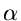(e)) with the path compression, where(e) is the inverse of an Ackerman function.
Example: See Figure 8.13.

E = {(1,3), (4,6), (2,5), (3,6), (3,4), (1,4), (2,3), (1,2), (3,5), (5,6) }Next:8.4 Traveling Salesman ProblemUp:8.3 Minimum-Cost Spanning TreesPrevious:8.3.2 Prim's Algorithm
eEL,CSA_Dept,IISc,Bangalore